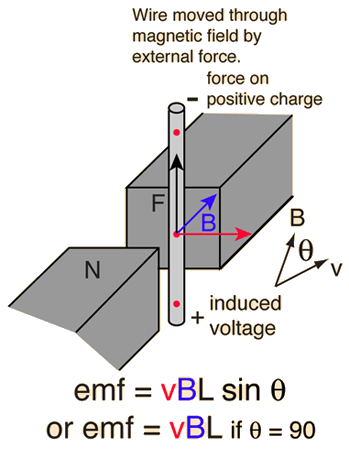# Voltage Generated in a Moving WireWhen a conductor moves through a magnetic field, there will be a generated motional emf. This is one example of Faraday's Law and it arises from the magnetic force. The voltage generated in a length of wire, presuming that the entire length moves through a uniform field, is given below.

 Magnetic interactions with charge
 Magnetic force applications

If the velocity is perpendicular to the magnetic field then the generated voltage is given by the simple product:

## Generated voltage = emf = Velocity x B-field x Length

For a wire of length L = m = x 10^ m
moving with velocity v= x 10^ m/s
perpendicular to a magnetic field B = Tesla = Gauss
the generated voltage is V = x 10^ V.
If the angle between the velocity and magnetic field is degrees
the generated voltage is V = x 10^ V.

Data may be entered in any of the fields. Whey you have finished entering data, click on the quantity you wish to calculate in the active formula above. The quantities will not be forced to be consistent until you click on a choice. Default values will be entered for unspecified parameters, but all values may be changed.

 How much current and power can you get from the generated voltage?
Index

Electromagnetic force

Magnetic field concepts

 HyperPhysics***** Electricity and Magnetism R Nave
Go Back